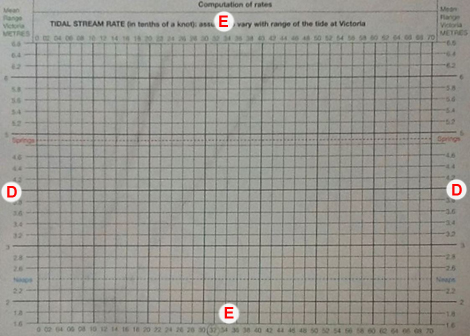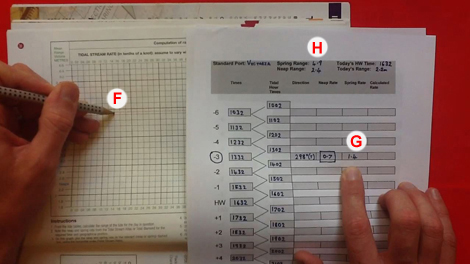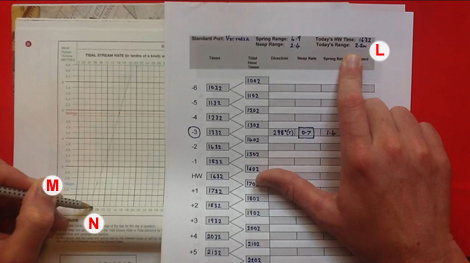# Tidal streams – YM

## Preparation

Before reading this page you may wish to read up on time zones and grab a copy of the training almanac (and a real one if possible).

## Why is this important?

At Yachtmaster level, we use a method that allows us to calculate the tidal stream as accurately as possible using the data available. It differs from the Day Skipper method only in the last step of calculating the tidal stream based on the current tide.

### Calculating the tidal stream rate

We are going to take the same example we looked at in the Dayskipper examples. Again Victoria is our standard port so the spring range is 4.9m and the neap range is 2.4m (point A). We have also calculated today’s range as 2.2m (point B). We also have the neap and spring rate of tidal flow (point C). All of this is the same as before.This time we are going to use a ‘computation of rates’ table to calculate as accurately as we can the rate (speed) of the tide on this particular day.

The scale on the left and right (points D) record to range of tide, the scale along the top and bottom (points E) record the rate of tidal stream.We first plot a point for the spring rate and range (point F). Across 1.4kt (spring rate – from point G) and up 4.9m (spring range – from point H).We secondly plot a point for the neap rate and range (point I). Across 0.7kt (neap rate – from point J) and up 2.4m (neap range – from point K).We draw a line with a ruler between these two points.We now use today’s tidal range of 2.2m (point L). We find 2.2m on the side scale of the table (point M) go across to meet the diagonal line and then down to find the tidal rate (point N).Therefore, when using this tidal stream to plot an Estimated Position or Course to Steer we will use:

– Direction: 298o(T)
– Rate: 0.6kt

Use this spreadsheet to check your calculations to Yachtmaster Tidal Stream questions. You can also use this to create and check questions of your own. Remember you will not be able to use this in the test.

To see the whole method explained watch this video.

### Extra help

Now watch this video from Motor Boat & yachtingThe following questions require the RYA Training Almanac and RYA Training Chart 3. See the sample answers below.

#### Question 1

Using tidal diamond M on Chart 3 calculate the effect of the tide on Sunday, 24 February between 0925 and 1025.

#### Question 2

Using tidal diamond M on Chart 3 calculate the effect of the tide on Sunday, 24 February between 1025 and 1225.

### Question 3

Using tidal diamond M on Chart 3 calculate the effect of the tide on Sunday, 24 February between 0805 and 0925.

### Question 4

Using tidal diamond M on Chart 3 calculate the effect of the tide on Sunday, 24 February between 0605 and 0805.

### Question 5

Using tidal diamond M on Chart 3 calculate the effect of the tide on Thursday, 11 April between 0605 and 0805.

Computation of Rates
Here is a guide from Cockpit Cards.

Tidal Streams
Learn how to calculate the tidal stream.

## Progression

You can now use you knowledge of tidal streams to help you navigate. Either, Estimated Position (to find out were you are) or Course to Steer (to find out which way you need to go).

## Feedback

The content of these pages is put together in good faith and is constantly evolving. It is possible that errors exist within this content. If you spot an error or would like to add anything to these pages please contact use via email.

Reading the content of these pages is not a substitute for completing a RYA Shorebased course or similar.